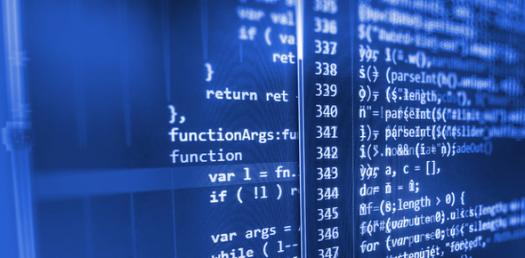# Introduction To Computer Science And Python Programming

40 Questions | Attempts: 431
ShareSettingsIntroduction to Computer Science and Python Programming

• 1.
What is the decimal value of the hexadecimal number 777?
• 2.
__________ is a way by which we can prevent data interception
• A.

Data decryption

• B.

Data Transmission

• C.

Data Encryption

• D.

Data Automation

• 3.
Which of the following statements is/are true?
• A.

A megabyte is roughly 1 million characters

• B.

A terabyte can store roughly 1 trillion characters

• C.

A terabyte can store more than just a few books

• D.

All of the above

• 4.
• 5.
• 6.
Data encryption is __________
• A.

A way by which we can decrypt data

• B.

The process of transforming data into an unintelligible form

• C.

Undoing the transformation of data at the point of reception

• D.

B & C

• 7.
________ was prominent in 5th generation computers
• A.

Large Scale Integration

• B.

Very Large Scale Integration

• C.

Extremely Large Scale Integration

• D.

A & B

• 8.
Which of the following statements is/are true?
• A.

A megabyte is roughly 1 million characters

• B.

A terabyte can store roughly 1 trillion characters

• C.

A&B

• D.

None of the above

• 9.
What is the approximate value of 453 kilobyte in megabyte?
• A.

453

• B.

0.453

• C.

4530

• D.

0.0453

• 10.
What is the result of the following hexadecimal arithmetic :10240 - 82A3
• A.

1F9D

• B.

843D

• C.

7F9D

• D.

743D

• 11.
What does an RGB value of (0, 0, 0) represent?
• A.

Black

• B.

White

• C.

Violet

• D.

Yellow

• 12.
Who is the first female professor of Computer Science in Africa?
• A.

• B.

• C.

Prof. Femi Osofisan

• D.

• 13.
The decision as to what data structures to use in implementing a system is best handled during ______
• A.

Program design

• B.

Maintenance

• C.

System design

• D.

Delivery

• 14.
One byte has ________ bits.
• A.

8

• B.

4

• C.

5

• D.

8

• 15.
A ___________ error does not cause the program to abort, but produces incorrect results.
• A.

Syntax

• B.

Runtime

• C.

Logic

• D.

Ende-time

• 16.
Which of these is not true of abstract data structures
• A.

A queue and a stack have two external pointers

• B.

Python supports dictionaries data structures

• C.

Python supports dictionaries data structures

• D.

A queue is a FIFO data structure

• 17.
What is the result of the statement:print ("1 + 3 * 2-5")
• A.

"1 + 3 * 2+5"

• B.

7

• C.

8

• D.

1 + 3 * 2-5

• 18.
Which of the followings is not an example of a linear data structure
• A.

List

• B.

Queue

• C.

Graph

• D.

Arrays

• 19.
Which of the following lines of code comes from a first generation computer language?
• A.

• B.

00010 1010 1101 0001 1010

• C.

This Total=TotalA + TotalC

• D.

Print("x > 0 and y > 0")

• 20.
The _____ operation says if and only if all inputs are 1, the output will be 1. The output will be 0 if any of the inputs are 0.
• A.

OR

• B.

NAND

• C.

NOR

• D.

AND

• 21.
What was the first truly mass auxiliary storage device?
• A.

Hard disk drive

• B.

Magnetic disk drive

• C.

Main memory drive

• D.

Magnetic tape drive

• 22.
Which of the options A to E gives the correct sequence for the software development methods listed below(i)Specification of needs (ii)Algorithm design (iii)Problem analysis (iv)Documentation (v)Testing and verification (vi)Implementation
• A.

I,ii,iii,iv,v,vi

• B.

I,iii,ii,v,vi,iv

• C.

I,iii,ii,vi,v,iv

• D.

I,ii,iii,v,vi,iv

• 23.
Suppose x = 1, y = -1, and z = 1. What will be displayed by the following statement?x = 1y = -1z = 1.if x > 0:  if y > 0:     print("x > 0 and y > 0")elif z > 0:  print("x < 0 and z > 0")
• A.

X > 0 and y > 0

• B.

X < 0 and z < 0

• C.

Nothing is displayed

• D.

The program has syntax error and won't run

• 24.
Analyze the following code.even = Trueif even = True:print("It is even!")
• A.

The program has syntax error in lines 1 and 2

• B.

The program has a syntax error in line 2

• C.

The program runs and displays True

• D.

The program runs and displays It is even!.

• 25.
Eliminating errors in a program is also called ______ the program
• A.

Debugging

• B.

Modularizing

• C.

Clarifying

• D.

All of the above

## Related TopicsBack to top
×

Wait!
Here's an interesting quiz for you.